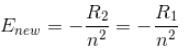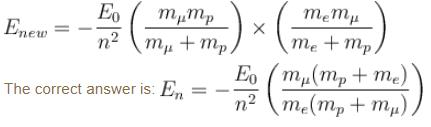Courses

# Origin Of Quantum Mechanics MCQ Level - 2

## 10 Questions MCQ Test Topic wise Tests for IIT JAM Physics | Origin Of Quantum Mechanics MCQ Level - 2

Description
This mock test of Origin Of Quantum Mechanics MCQ Level - 2 for Physics helps you for every Physics entrance exam. This contains 10 Multiple Choice Questions for Physics Origin Of Quantum Mechanics MCQ Level - 2 (mcq) to study with solutions a complete question bank. The solved questions answers in this Origin Of Quantum Mechanics MCQ Level - 2 quiz give you a good mix of easy questions and tough questions. Physics students definitely take this Origin Of Quantum Mechanics MCQ Level - 2 exercise for a better result in the exam. You can find other Origin Of Quantum Mechanics MCQ Level - 2 extra questions, long questions & short questions for Physics on EduRev as well by searching above.
QUESTION: 1

### An atom has n = 1 and n = 2 levels filled. How many electrons does the atom have?

Solution:

Number of electrons in nth orbit is given by 2n2
∴  total electrons is = 2(1)2 + 2(22)
= 2 + 8 = 10

QUESTION: 2

Solution:
QUESTION: 3

### de-Broglie hypothesized that the momentum and wavelength of a free massive particle are related by

Solution:

de-Broglie wave length, λ = h/p, where h is the Planck's constant.

The correct answer is: Planck’s constant

QUESTION: 4

Which of the following is true? When metallic surface is irradiated with white light

Solution:

White light does not produce any photoelectric effect because the range of wavelength in white light is (400 – 700)nm. The energy corresponding to this range of wavelength is small in comparison to the work function of any metal in general.

The correct answer is: No photo-current observed

QUESTION: 5

Positronium is an atom formed by an electron and a positron (anti electron), it is similar to the hydrogen atom with proton being replaced by a positron. If this positronium atom makes a transition from n = 3 to n = 1 the corresponding photon energy would be :

Solution:

The reduced mass of positronium is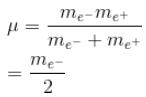In the case of hydrogen atom, for simplification we take the reduced mass of the electron and proton system to be me.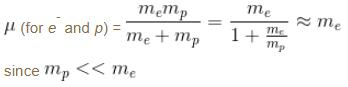So, the only difference between the hydrogen and positronium atom is that the positronium atom has the energy levels half of the hydrogen atom.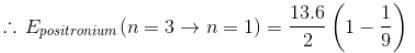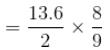= 6.0 eV
The correct answer is: 6.0 eV

QUESTION: 6

The energy required to remove both electrons from the helium atom in its ground state is 79.0 eV. How much energy is required to ionize helium? (i.e. to remove single electron)

Solution:

Consider He+ which is a hydrogen like atom. We can apply the Bohr theory to see that energy required to go from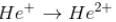What we need is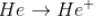Given is the total energy required to get He2+ from He.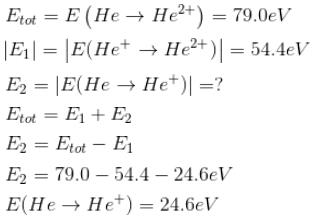We cannot apply Bohr theory directly to Helium because it is not a hydrogen like atom.

The correct answer is: 24.6 eV

QUESTION: 7

A particle with initial K.E = E and de-Broglie wavelength λ enters a region in which it has potential energy V. What is the particle’s new de-Broglie wavelength?

Solution:

In the initial case (free particle)
V = 0
Total energy = E = kinetic energy
Since the total energy remains conserved
∴ E = K.Enew + V
K.E. (new) = E – V
Now, new wavelength =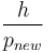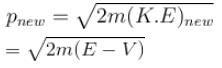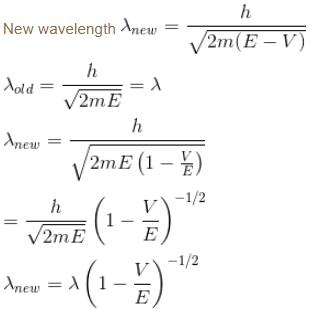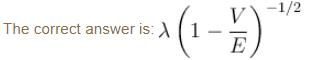QUESTION: 8

In an experimental observation of the photoelectric effect, the stopping potential was plotted against the frequency of the incident light. Which of the following is the slope of the line?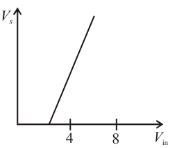Here h is the planck's constant and φ is the work function of the metal

Solution:

If Vs is the stopping potential then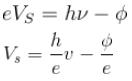Compare it with y = mx + c (equation of a straight time)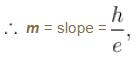c = intercept on y-axis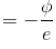QUESTION: 9

The energy from electromagnetic waves in equilibrium in a cavity is used to melt ice. If the temperature of the cavity is raised from T to 2T (in kelvin), then the mass of ice that can be melted increases by a factor of

Solution:

Energy radiated from a black body is proportional to the 4th power of its absolute temp is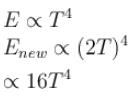Enew = 16E

Heat required to melt ice of mass m is proportional to the mass.

So, now the mass of ice that can be melted will 16 times the previous mass of ice melted.

QUESTION: 10

If we replaced the electron in a hydrogen atom with muon, (mass of a neon is 207 times the mass of an electron) then what will be the energy levels En of this new hydrogen atom in terms of the binding energy E0 (of the ordinary hydrogen), mass of the proton mp and the principal quantum number, n? (mµ = 207 me)

Solution:

In case the electron is replaced by the muon, we will have to consider the reduced mass of the proton- muon system.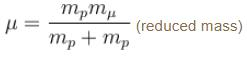The Rydberg constant is changed by a factor of.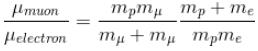The Rydbergs constant is proportional to the reduced mass of the system in consideration.

R1 = Rydbergs constant for e and p system is proportional to reduced mass of e and p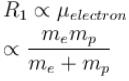R2 = Rydbergs constant for µ and p system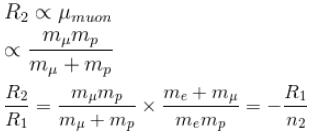∴  New energy levels are